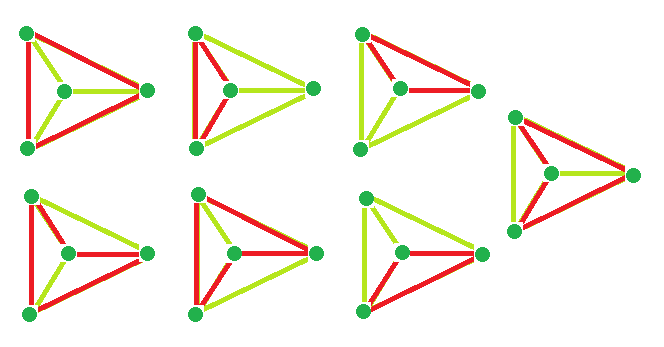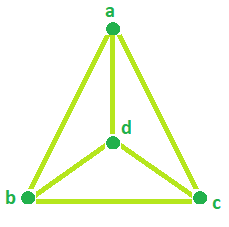# Program to find the diameter, cycles and edges of a Wheel Graph

Wheel Graph: A Wheel graph is a graph formed by connecting a single universal vertex to all vertices of a cycle. Properties:-

• Wheel graphs are Planar graphs.
• There is always a Hamiltonian cycle in the Wheel graph.
• Chromatic Number is 3 and 4, if n is odd and even respectively.

Problem Statement:
Given the Number of Vertices in a Wheel Graph. The task is to find:

1. The Number of Cycles in the Wheel Graph.
2. Number of edges in Wheel Graph.
3. The diameter of a Wheel Graph.
1. Examples:

```Input: vertices = 4
Output: Number of cycle = 7
Number of edge = 6
Diameter = 1

Input: vertices = 6
Output: Number of cycle = 21
Number of edge = 10
Diameter = 2

```

## Recommended: Please try your approach on {IDE} first, before moving on to the solution.

Example #1: For vertices = 4 Wheel Graph, total cycle is 7:Example #2: For vertices = 5 and 7 Wheel Graph Number of edges = 8 and 12 respectively:Example #3:For vertices = 4, the Diameter is 1 as We can go from any vertices to any vertices by covering only 1 edge.Formula to calculate the cycles, edges and diameter:-

```Number of Cycle = (vertices * vertices) - (3 * vertices) + 3
Number of edge = 2 * (vertices - 1)
Diameter = if vertices = 4, Diameter = 1
if vertices > 4, Diameter = 2
```

Below is the required implementation:

## C++

 `// C++ Program to find the diameter,  ` `// cycles and edges of a Wheel Graph ` `#include ` `using` `namespace` `std; ` ` `  `// Function that calculates the ` `// Number of Cycle in Wheel Graph. ` `int` `totalCycle(``int` `vertices) ` `{ ` `    ``int` `result = 0; ` ` `  `    ``// calculates no. of Cycle. ` `    ``result = ``pow``(vertices, 2) - (3 * vertices) + 3; ` ` `  `    ``return` `result; ` `} ` ` `  `// Function that calculates the ` `// Number of Edges in Wheel graph. ` `int` `Edges(``int` `vertices) ` `{ ` `    ``int` `result = 0; ` ` `  `    ``result = 2 * (vertices - 1); ` ` `  `    ``return` `result; ` `} ` ` `  `// Function that calculates the ` `// Diameter in Wheel Graph. ` `int` `Diameter(``int` `vertices) ` `{ ` `    ``int` `result = 0; ` ` `  `    ``// calculates Diameter. ` `    ``if` `(vertices == 4) ` `        ``result = 1; ` `    ``else` `        ``result = 2; ` ` `  `    ``return` `result; ` `} ` ` `  `// Driver Code ` `int` `main() ` `{ ` `    ``int` `vertices = 4; ` ` `  `    ``cout << ``"Number of Cycle = "` `<< totalCycle(vertices) << endl; ` `    ``cout << ``"Number of Edges = "` `<< Edges(vertices) << endl; ` `    ``cout << ``"Diameter = "` `<< Diameter(vertices); ` ` `  `    ``return` `0; ` `} `

## Java

 `//Java Program to find the diameter,  ` `// cycles and edges of a Wheel Graph ` `import` `java.io.*; ` ` `  `class` `GFG ` `{ ` `    ``// Function that calculates the  ` `    ``// Number of Cycle in Wheel Graph.  ` `    ``static` `int` `totalCycle(``double` `vertices)  ` `    ``{  ` `        ``double` `result = ``0``; ` `        ``int` `result1 = ``0``; ` `       `  `        ``// calculates no. of Cycle.  ` `        ``result = Math.pow(vertices, ``2``) - (``3` `* vertices) + ``3``;  ` `       `  `        ``result1 = (``int``)(result); ` `        ``return` `result1;  ` `    ``}  ` `       `  `    ``// Function that calculates the  ` `    ``// Number of Edges in Wheel graph.  ` `    ``static` `int` `Edges(``int` `vertices)  ` `    ``{  ` `        ``int` `result = ``0``;  ` `       `  `        ``result = ``2` `* (vertices - ``1``);  ` `       `  `        ``return` `result;  ` `    ``}  ` `       `  `    ``// Function that calculates the  ` `    ``// Diameter in Wheel Graph.  ` `    ``static` `int` `Diameter(``int` `vertices)  ` `    ``{  ` `        ``int` `result = ``0``;  ` `       `  `        ``// calculates Diameter.  ` `        ``if` `(vertices == ``4``)  ` `            ``result = ``1``;  ` `        ``else` `            ``result = ``2``;  ` `       `  `        ``return` `result;  ` `    ``} ` `     `  `    ``//Driver Code ` `    ``public` `static` `void` `main(String[] args) ` `    ``{ ` `        ``int` `vertices = ``4``; ` `         `  `        ``System.out.println(``"Number of Cycle = "` `+ totalCycle(vertices)); ` `        ``System.out.println(``"Number of Edges = "` `+ Edges(vertices)); ` `        ``System.out.println(``"Diameter = "` `+ Diameter(vertices)); ` `    ``} ` `} `

## Python3

 `# Python3 Program to find the diameter,  ` `# cycles and edges of a Wheel Graph  ` ` `  `# Function that calculates the  ` `# Number of Cycle in Wheel Graph.  ` `def` `totalCycle(vertices):  ` ` `  `    ``result ``=` `0` ` `  `    ``# calculates no. of Cycle.  ` `    ``result ``=` `(``pow``(vertices, ``2``) ``-`  `             ``(``3` `*` `vertices) ``+` `3``) ` `    ``return` `result  ` ` `  `# Function that calculates the  ` `# Number of Edges in Wheel graph.  ` `def` `Edges(vertices):  ` ` `  `    ``result ``=` `0` `    ``result ``=` `2` `*` `(vertices ``-` `1``)  ` `    ``return` `result  ` ` `  `# Function that calculates the  ` `# Diameter in Wheel Graph.  ` `def` `Diameter(vertices):  ` ` `  `    ``result ``=` `0` ` `  `    ``# calculates Diameter.  ` `    ``if` `vertices ``=``=` `4``:  ` `        ``result ``=` `1` `    ``else``: ` `        ``result ``=` `2` ` `  `    ``return` `result  ` ` `  `# Driver Code  ` `if` `__name__ ``=``=` `"__main__"``: ` ` `  `    ``vertices ``=` `4` ` `  `    ``print``(``"Number of Cycle ="``,  ` `           ``totalCycle(vertices))  ` `    ``print``(``"Number of Edges ="``, Edges(vertices))  ` `    ``print``(``"Diameter ="``, Diameter(vertices))  ` ` `  `# This code is contributed by Rituraj Jain `

## C#

 `// C# Program to find the diameter,  ` `// cycles and edges of a Wheel Graph ` `using` `System; ` `class` `GFG ` `{ ` `// Function that calculates the  ` `// Number of Cycle in Wheel Graph.  ` `static` `int` `totalCycle(``double` `vertices)  ` `{  ` `    ``double` `result = 0; ` `    ``int` `result1 = 0; ` ` `  `    ``// calculates no. of Cycle.  ` `    ``result = Math.Pow(vertices, 2) - ` `                     ``(3 * vertices) + 3;  ` ` `  `    ``result1 = (``int``)(result); ` `    ``return` `result1;  ` `}  ` ` `  `// Function that calculates the  ` `// Number of Edges in Wheel graph.  ` `static` `int` `Edges(``int` `vertices)  ` `{  ` `    ``int` `result = 0;  ` ` `  `    ``result = 2 * (vertices - 1);  ` ` `  `    ``return` `result;  ` `}  ` ` `  `// Function that calculates the  ` `// Diameter in Wheel Graph.  ` `static` `int` `Diameter(``int` `vertices)  ` `{  ` `    ``int` `result = 0;  ` ` `  `    ``// calculates Diameter.  ` `    ``if` `(vertices == 4)  ` `        ``result = 1;  ` `    ``else` `        ``result = 2;  ` ` `  `    ``return` `result;  ` `} ` ` `  `// Driver Code ` `public` `static` `void` `Main() ` `{ ` `    ``int` `vertices = 4; ` `     `  `    ``Console.WriteLine(``"Number of Cycle = "` `+  ` `                      ``totalCycle(vertices)); ` `    ``Console.WriteLine(``"Number of Edges = "` `+  ` `                           ``Edges(vertices)); ` `    ``Console.WriteLine(``"Diameter = "` `+  ` `                       ``Diameter(vertices)); ` `} ` `} ` ` `  `// This code is contributed by inder_verma `

## PHP

 ` `

Output:

```Number of Cycle = 7
Number of Edges = 6
Diameter = 1
```

Attention reader! Don’t stop learning now. Get hold of all the important DSA concepts with the DSA Self Paced Course at a student-friendly price and become industry ready.

My Personal Notes arrow_drop_upCheck out this Author's contributed articles.

If you like GeeksforGeeks and would like to contribute, you can also write an article using contribute.geeksforgeeks.org or mail your article to contribute@geeksforgeeks.org. See your article appearing on the GeeksforGeeks main page and help other Geeks.

Please Improve this article if you find anything incorrect by clicking on the "Improve Article" button below.